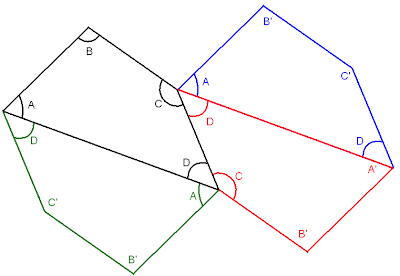## Saturday, September 25, 2010

### Why Tessellations are Geometrically Educational

I am really excited about the tessellations project I am in the middle of doing with some of my kids. It turns out that doing tessellationgs with a protractor and ruler is way more geometrically intense than simply learning how to accurately use a protractor.

Take a look at a "simple" tessellation below of a triangle. As it turns out, triangles get tessellated by forming parallelograms. In order to construct subsequent segments that are parallel to segments already existing, you need to look for and measure alternate interior angles to be congruent. In the picture below, I marked the original triangle in black, and the second triangle in red, the third triangle in green, the fourth in blue. Angles marked with an apostrophe (A') indicate angles that turn out to mathamagically be congruent to original angles WITHOUT the kids needing to measure them. (This is obvious by either the Third Angle Theorem or ASA.)It's pretty AWESOME how even the simplest tiling construction involves a series of geometric theorems that we can discuss later. And also awesome that these subsequent triangles are constructed without the use of a ruler (just straight edge and protractor).

The math is even juicier for tessellating quadrilaterals. As it turns out, quadrilaterals can be tiled by forming hexagons whose opposite sides are parallel and congruent. (Not unlike parallelograms.) In order to construct the new quadrilaterals, it turns out that we still measure only two pairs of alternate interior angles, but we also have to use a ruler to make sure the resulting parallel sides are also congruent. By some sort of SASAS rule (is that actually a theorem in introductory geometry?), the resulting quadrilateral will be congruent to the one you started off with. Again, in the diagram, A', B', and C' are angles that were not measured in the construction of those new quadrilaterals. They were mathamagically natural results of other parts of the construction!Truly phenomenal. I am thoroughly impressed by how something that seems so simple is brimming with math content.